# STATS 10 x Revision CONTENT COVERED CHAPTERS 1

• Slides: 32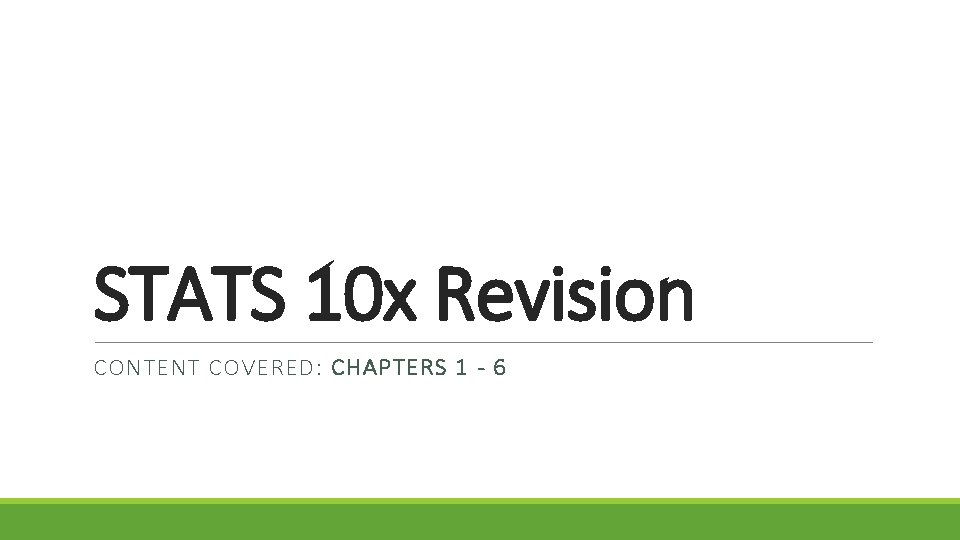STATS 10 x Revision CONTENT COVERED: CHAPTERS 1 - 6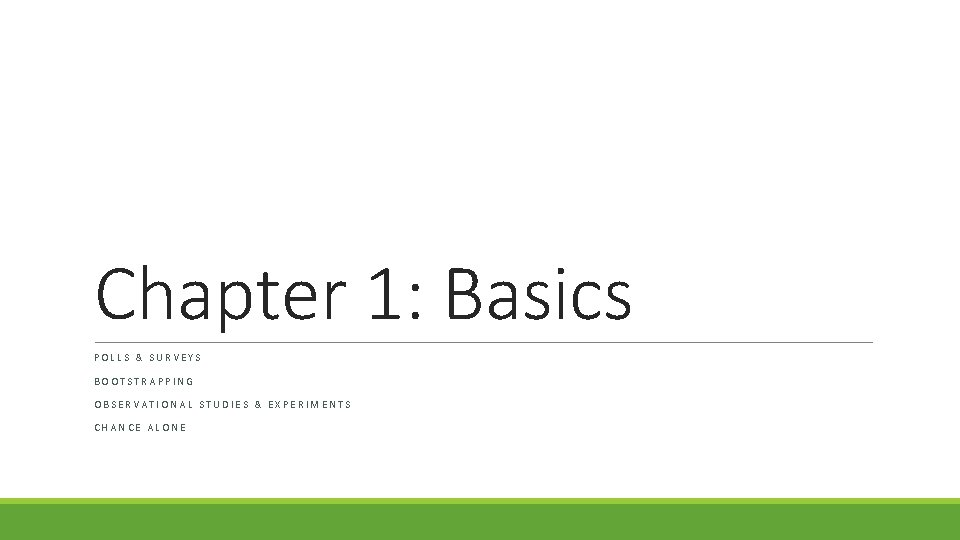Chapter 1: Basics POLLS & SURVEYS BOOTSTRAPPING OBSERVATIONAL STUDIES & EXPERIMENTS CHANCE ALONE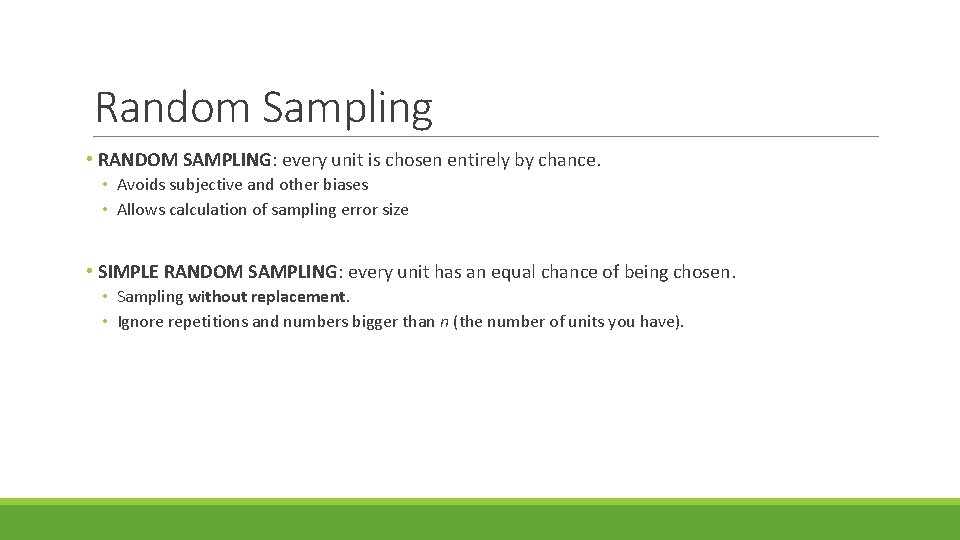Random Sampling • RANDOM SAMPLING: every unit is chosen entirely by chance. • Avoids subjective and other biases • Allows calculation of sampling error size • SIMPLE RANDOM SAMPLING: every unit has an equal chance of being chosen. • Sampling without replacement. • Ignore repetitions and numbers bigger than n (the number of units you have).Sampling Errors • “the price we pay for using a sample” over a census. • unavoidable. • might be bigger in smaller samples than larger samples. • size can be calculated.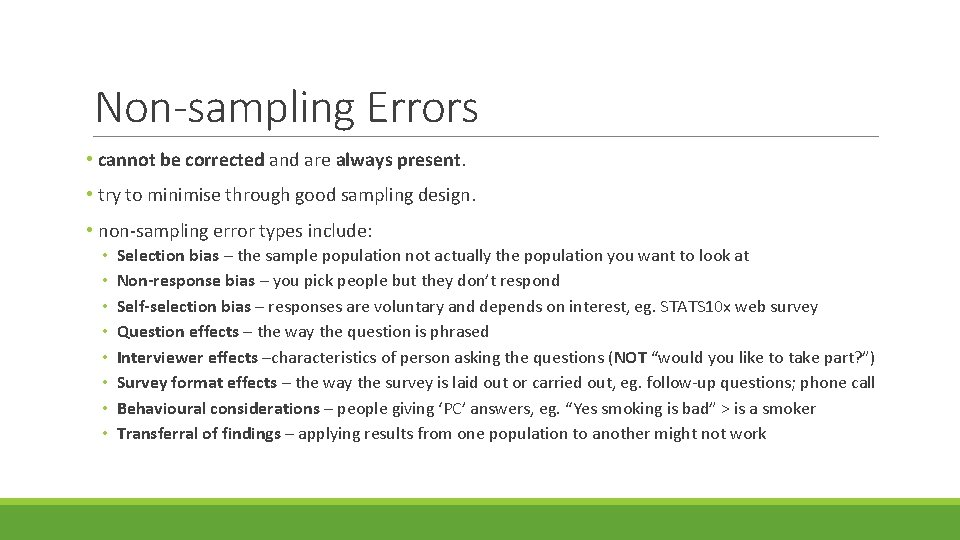Non-sampling Errors • cannot be corrected and are always present. • try to minimise through good sampling design. • non-sampling error types include: • • Selection bias – the sample population not actually the population you want to look at Non-response bias – you pick people but they don’t respond Self-selection bias – responses are voluntary and depends on interest, eg. STATS 10 x web survey Question effects – the way the question is phrased Interviewer effects –characteristics of person asking the questions (NOT “would you like to take part? ”) Survey format effects – the way the survey is laid out or carried out, eg. follow-up questions; phone call Behavioural considerations – people giving ‘PC’ answers, eg. “Yes smoking is bad” > is a smoker Transferral of findings – applying results from one population to another might not workBuilding Interval Estimates • Population: the group you want to find out about • Parameter: the characteristic you want to find out, eg. mean height of male STATS 10 x students • Always write parameters as μ, μ 1 – μ 2, P, P 1 – P 2 • Estimate: a known quantity from sample data to estimate the unknown parameter, eg. sample mean height of male STATS 10 x students • Always write estimates as x , p , etc. • Statistical Inference: process of using estimates to make useful information about a population, eg. applying the estimate confidence interval from sample of males to population of males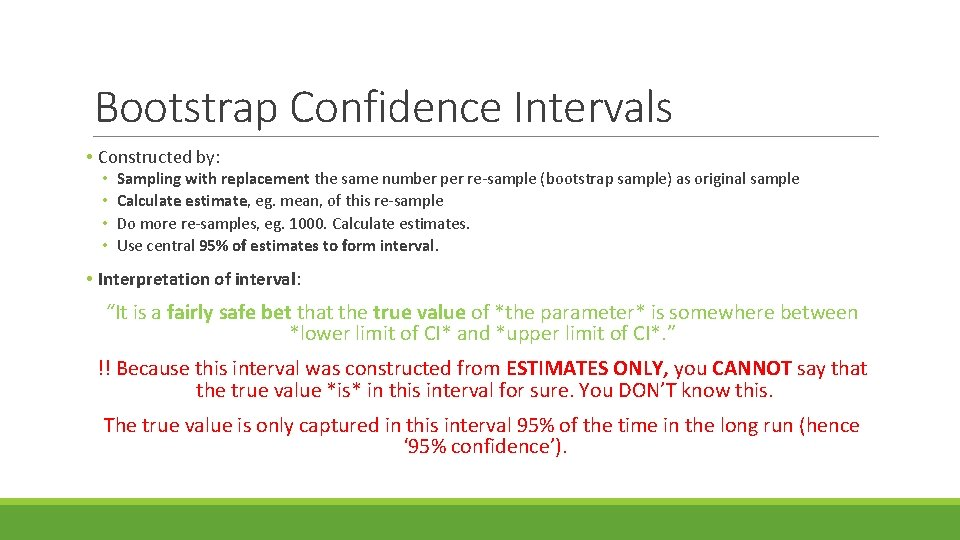Bootstrap Confidence Intervals • Constructed by: • • Sampling with replacement the same number per re-sample (bootstrap sample) as original sample Calculate estimate, eg. mean, of this re-sample Do more re-samples, eg. 1000. Calculate estimates. Use central 95% of estimates to form interval. • Interpretation of interval: “It is a fairly safe bet that the true value of *the parameter* is somewhere between *lower limit of CI* and *upper limit of CI*. ” !! Because this interval was constructed from ESTIMATES ONLY, you CANNOT say that the true value *is* in this interval for sure. You DON’T know this. The true value is only captured in this interval 95% of the time in the long run (hence ‘ 95% confidence’).Observational Study vs Experiment • OBSERVATIONAL STUDY: no treatment determined and imposed on units. • Cross-sectional: a ‘snapshot’ of a point in time • Longitudinal: over a long period of time, a series of cross-sectional studies. • EXPERIMENT: experimenter determines which units receive which treatment to be imposed. • Completely Randomised: treatments allocated entirely by chance to units. • Randomised Block: grouping units by a known factor (‘block’) then randomising. Examples of blocks could be age or gender. • Blinding / Double Blinding: subjects / subjects and experimenters don’t know treatment being imposed • Placebo: ‘dummy’ treatment • Placebo effect: response in humans when they believe they have been treated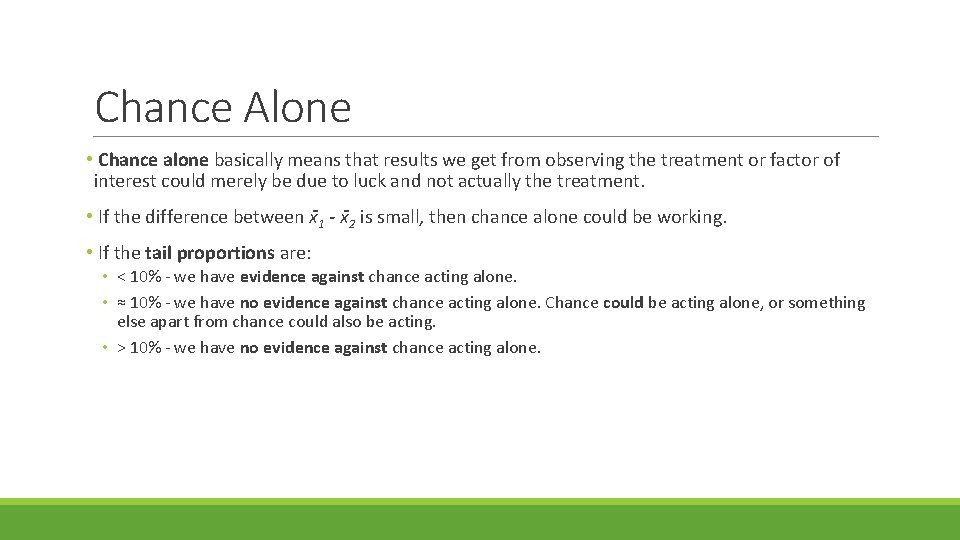Chance Alone • Chance alone basically means that results we get from observing the treatment or factor of interest could merely be due to luck and not actually the treatment. • If the difference between x 1 - x 2 is small, then chance alone could be working. • If the tail proportions are: • < 10% - we have evidence against chance acting alone. • ≈ 10% - we have no evidence against chance acting alone. Chance could be acting alone, or something else apart from chance could also be acting. • > 10% - we have no evidence against chance acting alone.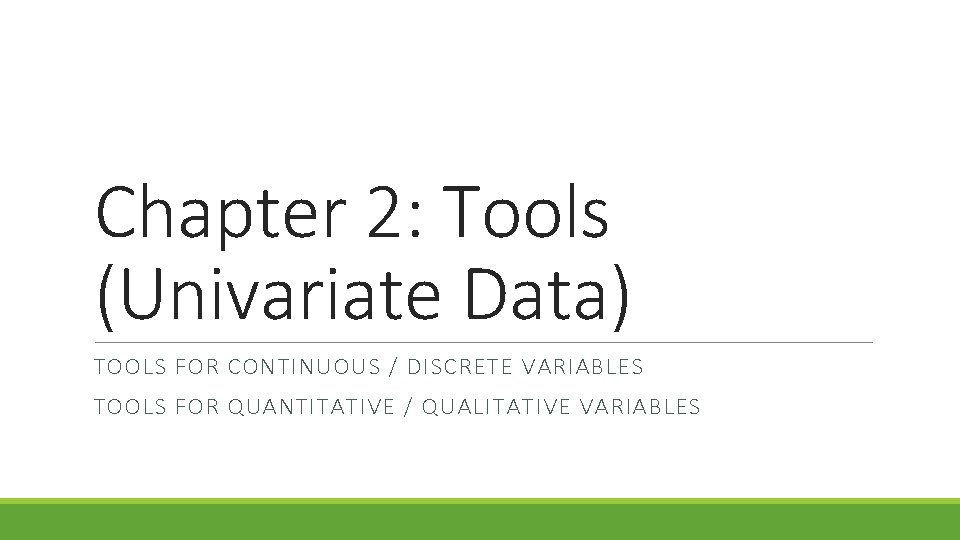Chapter 2: Tools (Univariate Data) TOOLS FOR CONTINUOUS / DISCRETE VARIABLES TOOLS FOR QUANTITATIVE / QUALITATIVE VARIABLESTools: Continuous Data The best indicator of which plot to use is SAMPLE SIZE. • DOT PLOT: ideal for small (< 20) samples. Shows clusters, groups and outliers. • STEM AND LEAF: ideal for medium (15 < n < 150) samples. Not good for large data sets. Shows density, shape of distribution and outliers. • BOX PLOT: ideal for moderate to large (> 30) samples. Good for comparing data sets. Shows centre, spread, skewness and outliers. No modality. • HISTOGRAM: ideal for large (> 50) samples. Shows density and distribution.Tools: Discrete Data • FREQUENCY TABLE: shows value and frequency of value occurrence. Sometimes has percentage columns. • BAR GRAPHS: shows frequency of value occurrence, similar to histogram (see previous slide). Shows density and distribution. Your values always go along the bottom (x) axis, and your frequency along the side (y) axis. Always list your values before your frequencies on tables.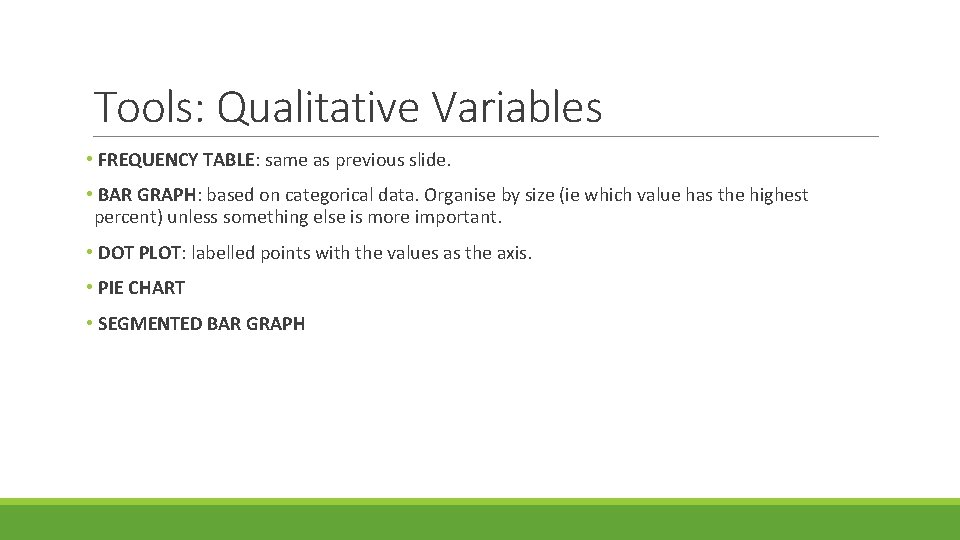Tools: Qualitative Variables • FREQUENCY TABLE: same as previous slide. • BAR GRAPH: based on categorical data. Organise by size (ie which value has the highest percent) unless something else is more important. • DOT PLOT: labelled points with the values as the axis. • PIE CHART • SEGMENTED BAR GRAPH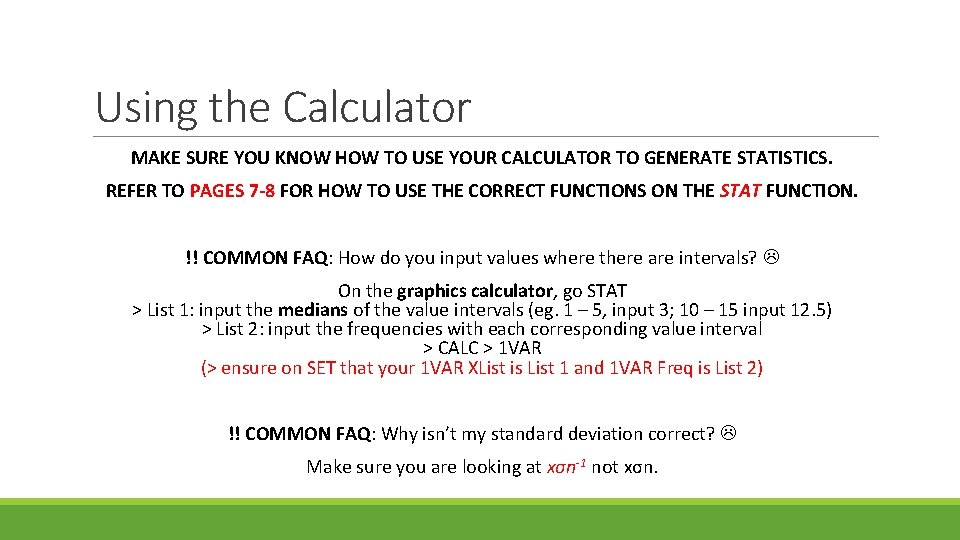Using the Calculator MAKE SURE YOU KNOW HOW TO USE YOUR CALCULATOR TO GENERATE STATISTICS. REFER TO PAGES 7 -8 FOR HOW TO USE THE CORRECT FUNCTIONS ON THE STAT FUNCTION. !! COMMON FAQ: How do you input values where there are intervals? On the graphics calculator, go STAT > List 1: input the medians of the value intervals (eg. 1 – 5, input 3; 10 – 15 input 12. 5) > List 2: input the frequencies with each corresponding value interval > CALC > 1 VAR (> ensure on SET that your 1 VAR XList is List 1 and 1 VAR Freq is List 2) !! COMMON FAQ: Why isn’t my standard deviation correct? Make sure you are looking at xσn-1 not xσn.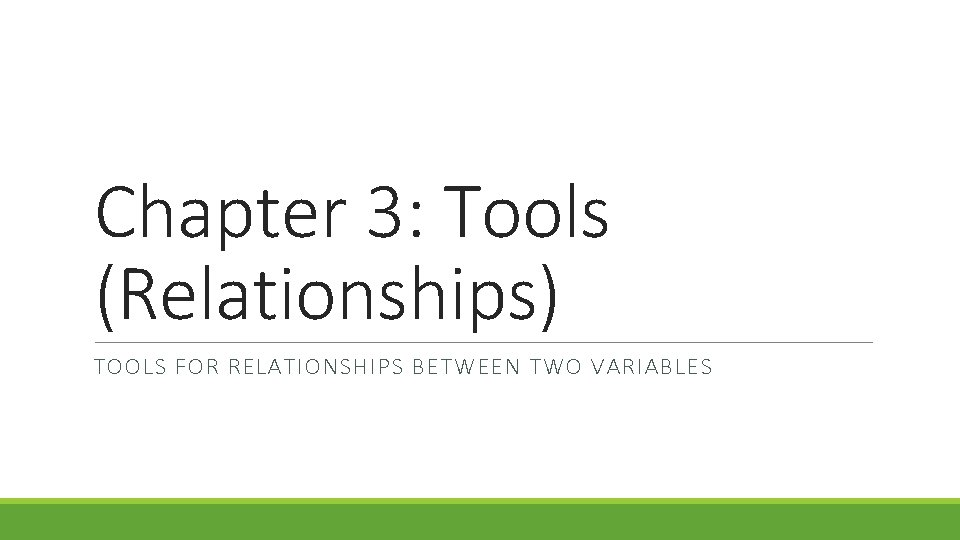Chapter 3: Tools (Relationships) TOOLS FOR RELATIONSHIPS BETWEEN TWO VARIABLES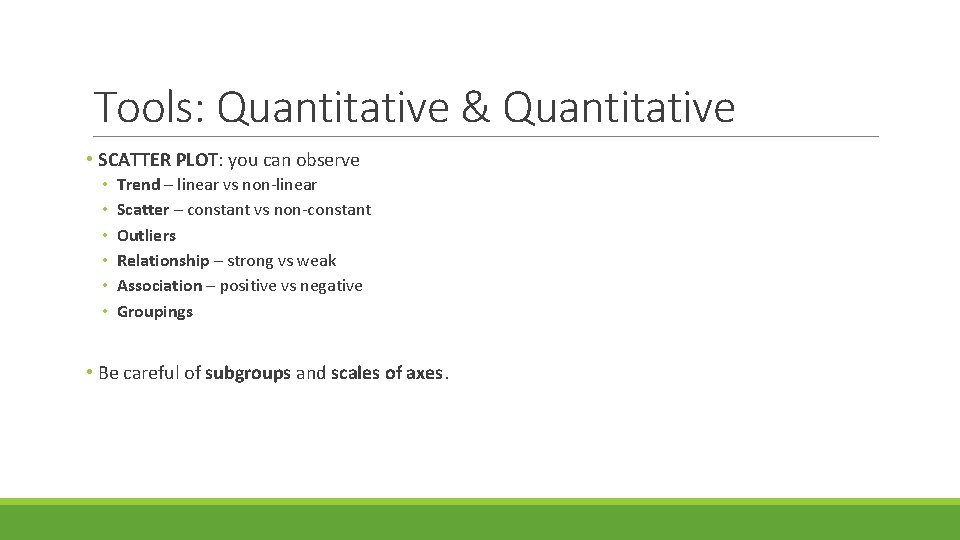Tools: Quantitative & Quantitative • SCATTER PLOT: you can observe • • • Trend – linear vs non-linear Scatter – constant vs non-constant Outliers Relationship – strong vs weak Association – positive vs negative Groupings • Be careful of subgroups and scales of axes.Tools: Quantitative & Qualitative • SIDE-BY-SIDE DOT OR BOX PLOT: you can observe differences in • • • Averages – eg. means Spread – range and variability Skewness Modality Individual group details such as outliers, clusters, groupings.Tools: Qualitative & Qualitative • TWO-WAY TABLE OF COUNTS: you can see frequencies, common vs uncommon combinations • BAR GRAPH OF PROPORTIONS: you can see common vs uncommon combinations, differences distributions and possibly modalities.Chapter 4: Probabilities and Proportions SIMPLE / JOINT / CONDITIONAL PROBABILITIES EVENT INDEPENDENCE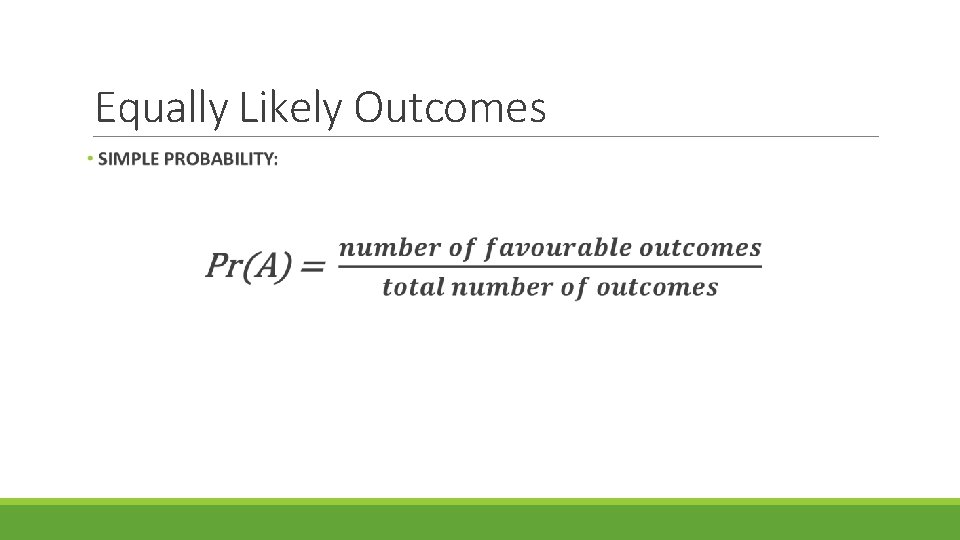Equally Likely OutcomesConditional Probability Event happening Conditional eventStatistical Independence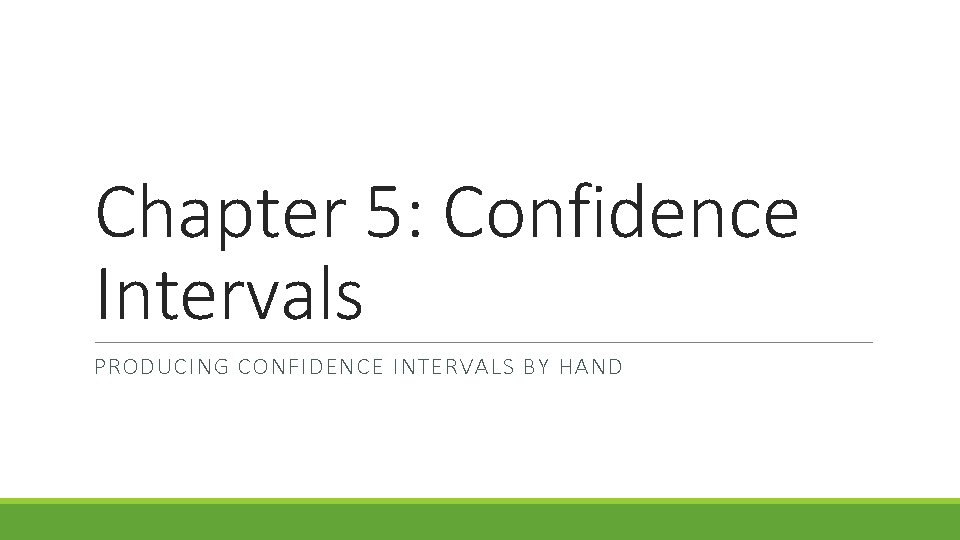Chapter 5: Confidence Intervals PRODUCING CONFIDENCE INTERVALS BY HAND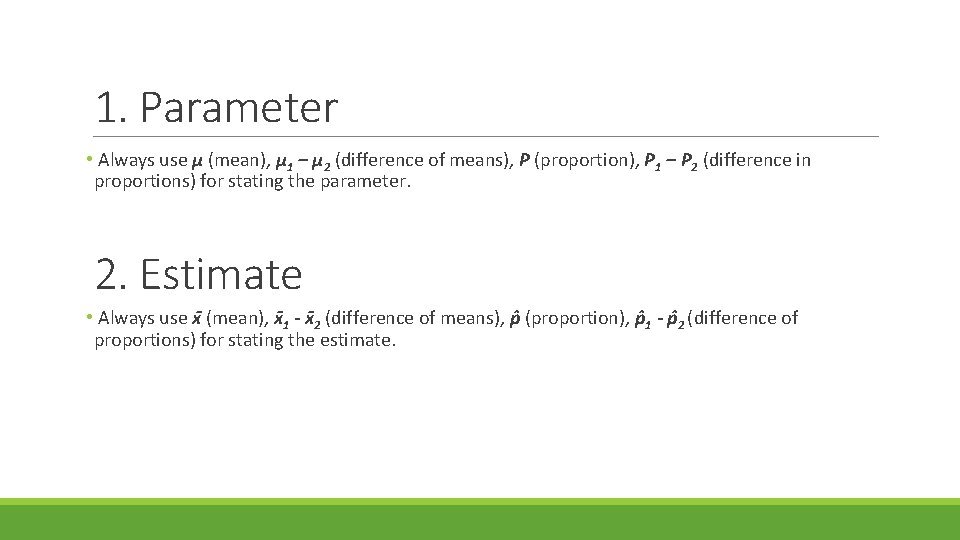1. Parameter • Always use μ (mean), μ 1 – μ 2 (difference of means), P (proportion), P 1 – P 2 (difference in proportions) for stating the parameter. 2. Estimate • Always use x (mean), x 1 - x 2 (difference of means), p (proportion), p 1 - p 2 (difference of proportions) for stating the estimate.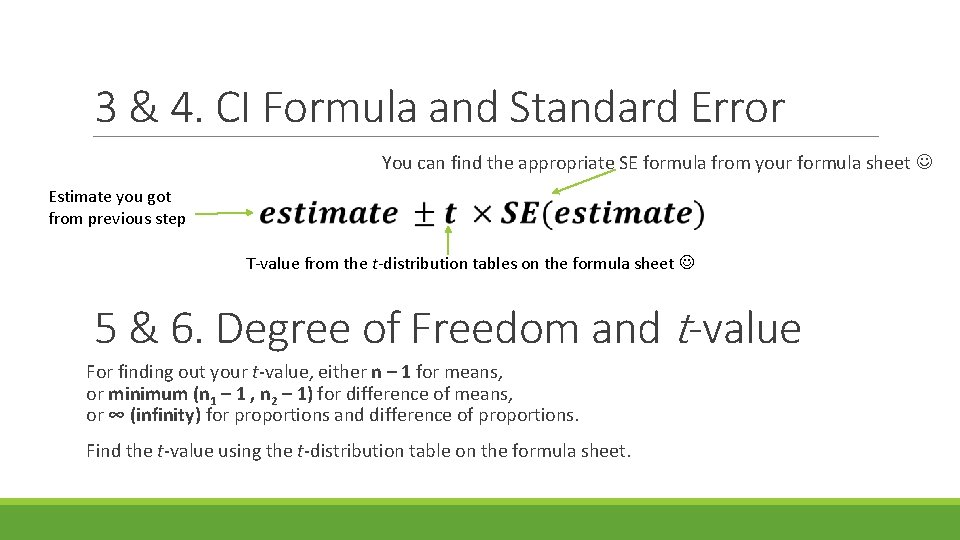3 & 4. CI Formula and Standard Error You can find the appropriate SE formula from your formula sheet Estimate you got from previous step T-value from the t-distribution tables on the formula sheet 5 & 6. Degree of Freedom and t-value For finding out your t-value, either n – 1 for means, or minimum (n 1 – 1 , n 2 – 1) for difference of means, or ∞ (infinity) for proportions and difference of proportions. Find the t-value using the t-distribution table on the formula sheet.7. Calculate the CI Limits Use the formula you wrote before, now filled with your estimate, t-value and standard error: 8. Interpretation “For *population of interest*, we can estimate with 95% confidence that *parameter of interest* is somewhere between *lower limit of CI* and *upper limit of CI*. ”Chapter 6: Hypothesis Testing HYPOTHESES T-TEST STATISTIC P-VALUE PRACTICAL VS STATISTICAL SIGNIFICANCEThe Null Hypothesis • The null hypothesis normally states there is ‘no difference’ or that there is ‘no effect’ of a treatment or factor of interest on the results. • Often it can be written as: NOTE: the hypothesised difference does not always have to be 0! Check the scenarios carefully Don’t forget to always write hypotheses with μ, μ 1 – μ 2, P, or P 1 – P 2 !The Alternative Hypothesis • The hypothesis you might favour while rejecting the null. It suggests that there is an effect on the results from the factor of interest. • It can be either one-sided or two-sided, which will affect your p-value later on. • A ONE-SIDED alternative hypothesis uses either a > or <, like this: • A TWO-SIDED alternative hypothesis uses an “is not equal to” sign instead of > or <, like this: Don’t forget to always write hypotheses with μ, μ 1 – μ 2, P, or P 1 – P 2 !The t-test Statistic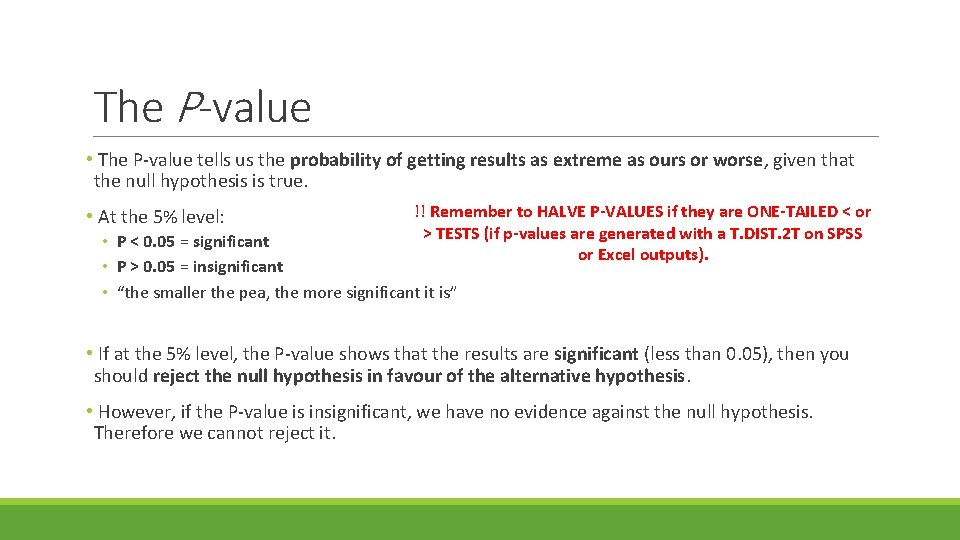The P-value • The P-value tells us the probability of getting results as extreme as ours or worse, given that the null hypothesis is true. • At the 5% level: !! Remember to HALVE P-VALUES if they are ONE-TAILED < or > TESTS (if p-values are generated with a T. DIST. 2 T on SPSS or Excel outputs). • P < 0. 05 = significant • P > 0. 05 = insignificant • “the smaller the pea, the more significant it is” • If at the 5% level, the P-value shows that the results are significant (less than 0. 05), then you should reject the null hypothesis in favour of the alternative hypothesis. • However, if the P-value is insignificant, we have no evidence against the null hypothesis. Therefore we cannot reject it.Statistical vs Practical Significance • Statistical significance can be argued through the interpretation of the P-value. • A statistically significant result has a P-value of less than 0. 05 (see previous slide). • Practical significance can be argued in relation to the effect size. It depends on the study’s context and scenario. • An example where practical significance is of greater significance could be in medication, where 1 mg could make a huge difference in effects on a patient, but the P-value may suggest otherwise. • However, in a different context, 1 mg of sugar per lollipop may not be of practical significance. • Further examples outlining when practical significance is or is not important can be found in the Coursebook, Chapter 6, page 12.